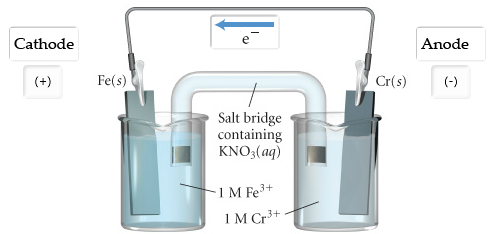# Problem: Write a balanced equation for the overall reaction. Express your answer as a chemical equation. Identify all of the phases in your answer. Calculate E°cell. Express your answer using two significant figures.

###### FREE Expert Solution

 Anode is in the negative side (left side of the diagram)

▪ Anode → oxidation

oxidation half-reaction happens in the anode solution

▪ losing electrons → becoming more positive → anode dissolves away

 Cathode is in the positive side (right side of the diagram)

▪ Cathode → reduction

reduction half-reaction happens in the anode solution

▪ gaining electrons → becoming more negative → solids is deposited in the surface

91% (479 ratings)###### Problem DetailsWrite a balanced equation for the overall reaction. Express your answer as a chemical equation. Identify all of the phases in your answer.